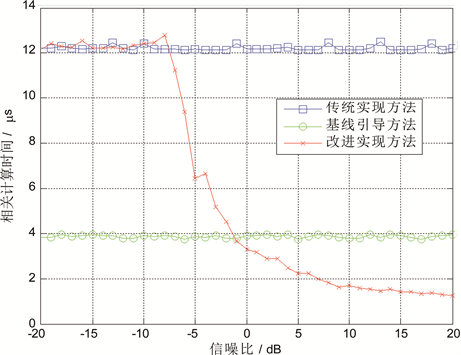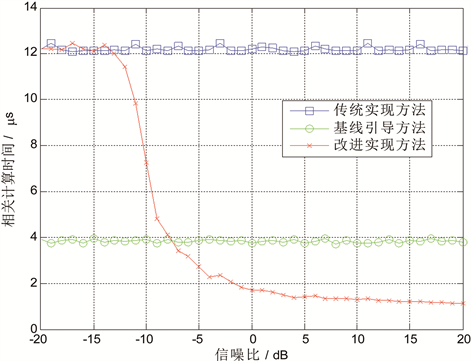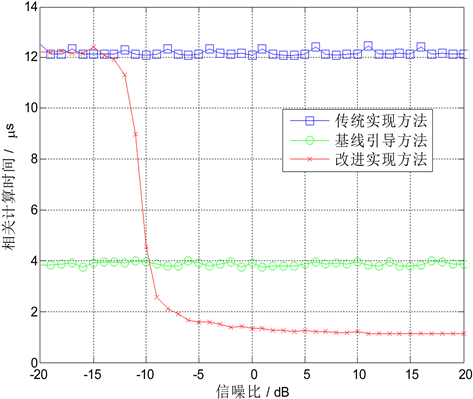﻿ 一种相关干涉仪测向算法的快速实现

# 一种相关干涉仪测向算法的快速实现A Fast Implementation of Correlative Interferometry for Direction-Finding

Abstract: As one of the most widely adopted direction-finding (DF) techniques, correlative interferometry (CI) suffers from its efficiency problem occasionally, especially when DF results are required in real time, since the original CI method is based on massive computation of vector correlations. Herein a fast implementation of the CI technique is proposed. Based on the correlation coefficient of the sequential measured phase errors, the implementation reduces the range of available samples and cuts the number of computation of correlations down. Simulations have demonstrated the efficacy of the proposed method over the original one.

1. 引言

2. 相关干涉仪算法

$\Phi =\left[\begin{array}{cccc}{\phi }_{11}& {\phi }_{12}& \cdots & {\phi }_{1N}\\ {\phi }_{21}& {\phi }_{22}& \cdots & {\phi }_{2N}\\ ⋮& ⋮& \ddots & ⋮\\ {\phi }_{M1}& {\phi }_{M2}& \cdots & {\phi }_{MN}\end{array}\right]=\left[{\phi }_{1},{\phi }_{2},\cdots ,{\phi }_{N}\right]$ (1)

$\mathbb{I}=\left\{i|1\le i\le N,\text{\hspace{0.17em}}i\in ℤ\right\}$ (2)

${\phi }_{i}={\left[{\phi }_{1i},{\phi }_{2i},\cdots ,{\phi }_{Mi}\right]}^{\text{T}},i\in \mathbb{I}$ (3)

$\stackrel{^}{\phi }={\left[{\phi }_{1},{\phi }_{2},\cdots ,{\phi }_{M}\right]}^{\text{T}}$ (4)

${\rho }_{i}=\frac{|{\phi }_{i}^{\text{H}}\stackrel{^}{\phi }|}{‖{\phi }_{i}‖\cdot ‖\stackrel{^}{\phi }‖}$ (5)

${\rho }_{i}$ 的最大值的索引i对应的是测得的角度。

3. 测向算法快速实现

3.1. 方法推导

${\rho }_{i}=\mathrm{cos}\left[\alpha \left(\stackrel{^}{\phi },{\phi }_{i}\right)\right]$ (6)

${\rho }_{i}$ 取最大值时的角度索引为 ${i}_{\mathrm{max}}$ ，对应的矢量夹角为

${\alpha }_{\mathrm{min}}=\alpha \left(\stackrel{^}{\phi },{\phi }_{{i}_{\mathrm{max}}}\right)$ (7)

${\alpha }_{\mathrm{min}}+\text{2}×\alpha \left(\stackrel{^}{\phi },{\stackrel{^}{\phi }}^{\prime }\right)<\text{π}/2$ (8)

${\rho }_{\text{th}}=\mathrm{cos}\left[{\alpha }_{\mathrm{min}}+\text{2}×\alpha \left(\stackrel{^}{\phi },{\stackrel{^}{\phi }}^{\prime }\right)\right]$ (9)

$\mathbb{K}=\left\{k|{\rho }_{k}\ge {\rho }_{\text{th}},\text{\hspace{0.17em}}k\in \mathbb{I}\right\}$ (10)

$\alpha \left(\stackrel{^}{\phi },{\phi }_{h}\right)>{\alpha }_{\mathrm{min}}+2×\alpha \left(\stackrel{^}{\phi },{\stackrel{^}{\phi }}^{\prime }\right)$ (11)

$\alpha \left({\stackrel{^}{\phi }}^{\prime },{\phi }_{h}\right)\ge \alpha \left(\stackrel{^}{\phi },{\phi }_{h}\right)-\alpha \left(\stackrel{^}{\phi },{\stackrel{^}{\phi }}^{\prime }\right)>{\alpha }_{\mathrm{min}}+2×\alpha \left(\stackrel{^}{\phi },{\stackrel{^}{\phi }}^{\prime }\right)-\alpha \left(\stackrel{^}{\phi },{\stackrel{^}{\phi }}^{\prime }\right)>{\alpha }_{\mathrm{min}}+\alpha \left(\stackrel{^}{\phi },{\stackrel{^}{\phi }}^{\prime }\right)$ (12)

$\begin{array}{c}\alpha \left({\stackrel{^}{\phi }}^{\prime },{\phi }_{{i}_{\mathrm{max}}}\right)\le \alpha \left(\stackrel{^}{\phi },{\phi }_{{i}_{\mathrm{max}}}\right)+\alpha \left(\stackrel{^}{\phi },{\stackrel{^}{\phi }}^{\prime }\right)\\ \le {\alpha }_{\mathrm{min}}+\alpha \left(\stackrel{^}{\phi },{\stackrel{^}{\phi }}^{\prime }\right)\end{array}$ (13)

$\alpha \left({\stackrel{^}{\phi }}^{\prime },{\phi }_{h}\right)>\alpha \left({\stackrel{^}{\phi }}^{\prime },{\phi }_{{i}_{\mathrm{max}}}\right)$ (14)

${\stackrel{^}{\phi }}^{\prime }$${\phi }_{h}$ 的相关系数小于 ${\stackrel{^}{\phi }}^{\prime }$${\phi }_{{i}_{\mathrm{max}}}$ 的相关系数，因此得出结论，在 $\Phi$ 中与 ${\stackrel{^}{\phi }}^{\prime }$ 相关系数最大的角度索引必定是集合 $\mathbb{K}$ 的元素，所以在对 ${\stackrel{^}{\phi }}^{\prime }$ 进行测向时，只需要与 $\mathbb{K}$ 中所有角度索引的样本做相关运算和峰值搜索即可。

3.2. 实现流程

· 1) 首次进行测向，将采集的相位差矢量 $\stackrel{^}{\phi }$ 与样本库中所有角度的样本进行相关计算，得到 ${\rho }_{i}$

· 2) 搜索 ${\rho }_{i}$ 中的最大值，得到本次测向的结果 ${i}_{\mathrm{max}}$ ，记录最大值对应的矢量夹角 ${\alpha }_{\mathrm{min}}$

· 3) 进行下一次测向，计算采集的相位差矢量 ${\stackrel{^}{\phi }}^{\prime }$$\stackrel{^}{\phi }$ 的夹角 $\alpha \left(\stackrel{^}{\phi },{\stackrel{^}{\phi }}^{\prime }\right)$

· 4) 如果不满足公式(8)，则重复步骤1)；

· 5) 如果满足公式(8)，则将按照式(9)计算相关系数门限，将 ${\rho }_{i}$ 中所有不小于 ${\rho }_{\text{th}}$ 的角度索引生成新的角度索引集合 $\mathbb{K}$

· 6) 将 ${\stackrel{^}{\phi }}^{\prime }$$\mathbb{K}$ 中所有角度的样本进行相关运算，并进行峰值搜索，得到测向结果；

· 7) 重复步骤3)，继续进行测向。

3.3. 方法仿真

$\Delta =\underset{{R}_{\text{SN}}=-20}{\overset{20}{\sum }}\underset{q=1}{\overset{1000000}{\sum }}|{i}_{q1}-{i}_{q2}|$ (15)Figure 1. Simulation result of computation time for 30 MHz frequencyFigure 2. Simulation result of computation time for 100 MHz frequencyFigure 3. Simulation result of computation time for 300 MHz frequency

4. 结论

 翟庆伟, 王玉, 宫兵. 波束比幅法测向技术研究[J]. 无线电通信技术, 2007, 33(6): 55-56.

 王帅, 张军. 基于到达时差测向法的目标角度测量技术研究[J]. 传感器世界, 2015, 11(21): 31-34.

 谢东. 干涉仪测向技术的研究[D]: [硕士学位论文]. 成都: 四川大学, 2003.

 陈旭彬, 任培明, 戴慧玲. 空间谱估计无线电测向系统[J]. 电信科学, 2017, 7(33): 183-188.

 杜政东, 魏平. 基线引导式快速相关干涉仪测向性能分析及提升方法[J]. 信号处理, 2016, 32(3): 327-334.

 龚军涛, 黄光明, 高由兵.一种改进的相关干涉仪测向算法[J]. 电子信息对抗技术, 2016, 31(1): 4-7.

 耿赟, 谷振宇. 基于FPGA的短波相关干涉仪测向[J]. 舰船电子对抗, 2014, 37(2): 57-60.

 王云龙, 吴瑛. 基于GPU的相关干涉仪算法实现[J]. 信息工程大学学报, 2015, 16(1): 41-45.

Top